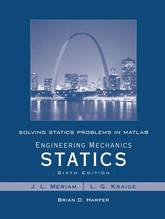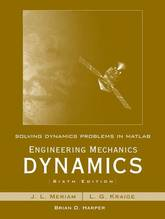Filter
(found 69 products)Learn MATLAB to help you understand mechanics MATLAB is a powerful tool for mathematical modeling, and is becoming essential to many engineering fields. Solving Statics Problems in MATLAB is designed to both introduce students to MATLAB, and provide them with a useful tool for understanding static mechanics. The book begins ...
Solving Statics Problems in MATLAB to accompany Engineering Mechanics Statics 6e
Learn MATLAB to help you understand mechanics MATLAB is a powerful tool for mathematical modeling, and is becoming essential to many engineering fields. Solving Statics Problems in MATLAB is designed to both introduce students to MATLAB, and provide them with a useful tool for understanding static mechanics. The book begins with MATLAB basics, including scripts, functions, graphics, calculations, and equations, then moves into physics with targeted guidance on solving force systems, equilibriums, structures, friction, and more. The problems presented are taken from Engineering Mechanics Statics, 6th Edition, but the clear guidance and comprehensive instruction can be applied to any course.
56.650000 USD

Solving Statics Problems in MATLAB to accompany Engineering Mechanics Statics 6e

by L. G. Kraige, J. L. Meriam, Brian Harper
Paperback / softbackAn introduction to MATLAB for engineering students, complete with practice problems Written as a complement to Engineering Mechanics Dynamics, this book provides students with an introduction to MATLAB as well as example problems that correspond to the aforementioned text. The book covers numerical calculations, defining functions, graphics, symbolic calculations, differentiation ...
Solving Dynamics Problems in MATLAB to accompany Engineering Mechanics Dynamics 6e
An introduction to MATLAB for engineering students, complete with practice problems Written as a complement to Engineering Mechanics Dynamics, this book provides students with an introduction to MATLAB as well as example problems that correspond to the aforementioned text. The book covers numerical calculations, defining functions, graphics, symbolic calculations, differentiation and integration, and solving equations with MATLAB and then presents problems in seven subsequent chapters. These cover kinematics of particles, kinetics of particles, kinetics of systems of particles; plane kinematics of rigid bodies; plane kinetics of rigid bodies, three-dimensional dynamics of rigid bodies, and vibration and response time.